Some polyhedral patterns

Three Golden Rectangles in an Icosahedron
 A "golden rectangle" is one with edge ratio 1:, where= (1+5)/2 = 1.618... is the famous "Golden Ratio" known since ancient times. The ratio of certain diagonals of an icosahedron to the edge length is:1 , which means that certain sets of four vertices form a golden rectangle. In fact, the twelve vertices can be partitioned into the corners of four golden rectangles. There are two ways of doing this, known as the "True cross" (left) and the "Skew cross" (right)... they have different symmetries. The symmetry of the left-hand example, with rectangle edges oriented parallel to the coordinate axes, gives a useful set of coordinates for the vertices of the icosahedron, as the 12 even permutations of <1,,0>. To find out more about these arrangements, you can look up H.S.M. Coxeter's paper "12 points in PG(3, 5) with 95040 self-transformations" in his 12 Geometrical Essays or John Baez' "Some thoughts on the number six". There is far more here than meets the eye!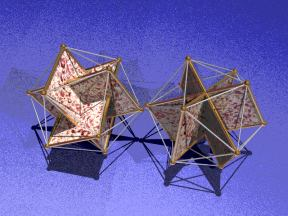Three Rectangles and a Cube in a Dodecahedron

Five Rectangles in a Dodecahedron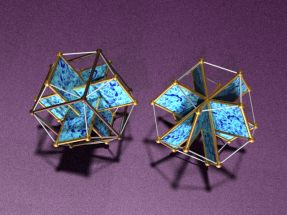The golden rectangle does not fit a dodecahedron, which has more vertices and consequently shorter edges. However, a rectangle whose edge ratio is 1:˛ does. The skew cross configuration generalizes (right); the true cross does not, but a cube (on the left;darker metal) and three of these rectangles can between them occupy all twenty vertices of a dodecahedron. Note the unexpected pattern that the cube makes with the facets of the dodecahedron, in which each of the twelve edges of the cube forms the diagonal of one of the dodecahedron's twelve facets . The left image, like the true cross above, suggests a useful set of coordinates for the twenty vertices of a dodecahedron: the twelve even permutations of <0,1,˛> together with the eight points <,,>.
A rhombic dodecahedron
 The rhombic dodecahedron is not a regular solid, because its facets are not regular, and it has three meeting at some vertices and four at others. This "paperweight" shows that each facet is directly above its opposite facet. The rhombic dodecahedron is an "Archimedean dual", That is, its vertices correspond to the facets of an "Archimedean solid", in this case the cuboctahedron. One of its interesting properties is that - like the cube, and unlike any other regular polyhedron - it can pack space. A set of blocks in this shape can fill a region of space with no cracks between them.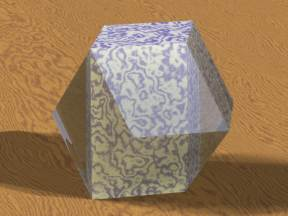A stella octangula inscribed in a hyperboloid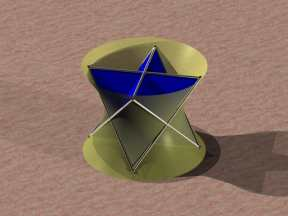The stella octangula is a compound of two tetrahedra. It can also be thought of as a star antiprism - one of a family of polyhedra in which two star polygons (here the degenerate 4/2; the pentagram is another example) are joined by triangles that join edges on one side to vertices on the other. Any antiprism has its "side edges" divided into two disjoint families that "twist" about the central axis with equal and opposite inclinations to the vertical; thus those edges lie completely within a single one-sheeted hyperboloid.
A crossed antiprism inscribed in a hyperboloid
 Another hyperboloid inscription, this time with a pentagrammatic crossed antiprism (the fifth one in the top row here .) This weird polyhedron has two pentagrams as top and bottom faces. The cener of one is above the center of the other, but the vertices are rotated 36 degrees so that seen from above they alternate. Then ten equilateral triangles are added, each one sharing an edge with two of the others and cutting the rest. Because the triangles lean over so much, the eges are heavily inclined and the resulting hyperboloid is very narrow-waisted.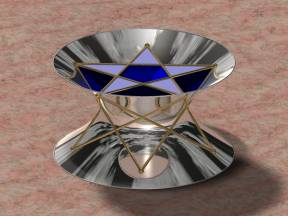In the Belly of the Grand Antiprism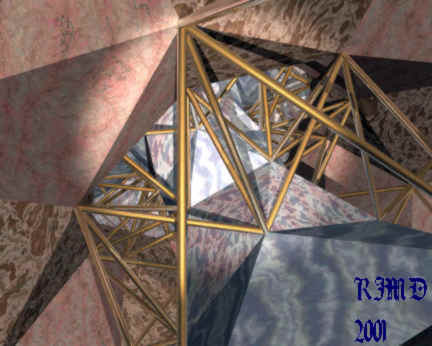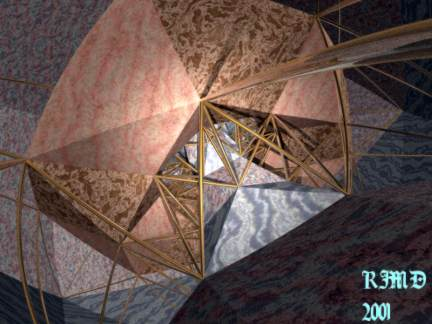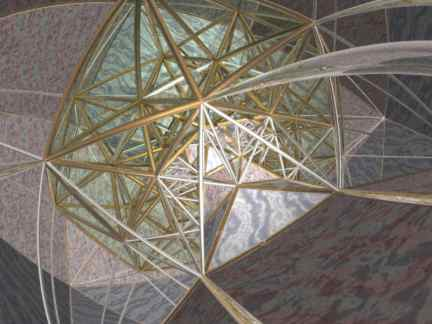One of the strangest polytopes in four dimensions is the Grand Antiprism discovered by Conway and Guy. It has 20 facets that are pentagonal antiprisms, joined like beads by their pentagonal facets into two rings of 10. These rings follow the great circles x2+y2 = 1, u=v=0 and u2+v2 = 1, x=y=0 on the hypersphere. First surprise: these are linked within the surface of the polytope (though not in all of R4: an analogy is the way in which the equator separates the North and South Poles on the surface ofthe earth, but not in three-space.) Then the space between them is filled with a "crust" of 300 tetrahedra: 100 with a triangular face on the first ring and a vertex on the second, 100 with an edge on each, and 100 with a vertex on the first and a face on the second. The Grand Antiprism is the only four-dimensional convex uniform polytope that cannot be obtained from Wythoff's construction.

Representing a four-dimensional polytope in a two-dimensional picture is difficult. One approach, used here, is to first map the polytope (topologically a 3-sphere) into R3 (ordinary three-space) by stereographic projection, dropping one point (or "sending it to infinity"). Then some cells - here the antiprisms - are represented as solid, and the rest by their edges, so one sees the faces as if from inside the faces (not inside the four-dimensional interior of the polyhedron.)

The second image uses an "ultra-wide-angle lens" to show more of the structure; the bending of edges arises from this and not from the stereographic projection. (In fact, I cheated: only the vertices were mapped into ordinary 3-space, and the edges and triangles between them are Euclidean. The apparent curvature is an artifact of the lens)

The Grand Antiprism is closely related to the 600-cell, in roughly the same way that the pentagonal antiprism is related to the icosahedron. If you slice two pentagonal prism caps ("Arctic and Antarctic regions") off the icosahedron, a pentagonal antiprism remains. Or, conversely, covering the pentagonal caps of the pentagonal antiprism with triangles gives an icosahedron. We can think of this as first filling the pentagons with triangles, then making those triangles equilateral so they stand up out of the plane of the pentagon.

Similarly, the two rings of antiprisms can be filled with non-regular tetrahedra - ten tetrahedra going in from the center of each antiprism to its center, and a lens-shaped ring of five joining the centers of each two adjacent antiprisms in the chain to each of their shared edges. Each ring of ten antiprisms is thus triangulated into 150 tetrahedra. Making those regular "puffs them out into the fourth dimension" and yields two interlocking equatorial belts which, together with the other 300 tetrahedra in between give the 600 cells of the 600-cell. The bottom picture here shows one of the rings triangulated in this way.

Note that while the rings of antiprisms can be divided into regular tetrahedra, the individual antiprisms cannot. Otherwise, we could omit every other antiprism in each ring to get another semiregular polytope.

An unusual polyhedron
These two images show an odd polyhedron related to the semilune tilings here. It has two sorts of face, a scalene triangle and a "bat-shaped" crossed quadrilateral. All its vertices lie on a sphere. In the upper picture, both types of faces are shown opaque, which emphasizes the triangular faces; in the lower picture the triangles are transparent which makes the "bats" easier to see. The odest feature of the polyhedron is that half of each crossed "bat" face faces one way, and half faces the other way.

The corresponding spherical tiling is obtained by projecting the faces and edges out onto the sphere. It only has one sort of face, the triangles. Where do the "bats" go? In the polyhedron, they lie in planes passing through the center of the sphere; so they project edge-on into great semicircles. The only sign of their existence in the spherical tiling is the non-edge-to-edge meeting between the triangles. Any other such non-edge-to-edge tiling of the sphere also corresponds to an edge-to-edge polyhedron with edge-on crossed polygons replacing the broken edges.

There are other polyhedra with edge-on faces, for instance the tetrahemihexahedron which is obtained by letting three squares intersect like an octahedral radar reflector (each two orthogonal and sharing a diagonal) and then capping half the eight "pits" with triangles. The resulting polyhedron is nonconvex, nonorientable like a Klein bottle, and has seven faces - three square and four triangular.

In contrast, the polyhedron shown here is orientable. We can either think of the "bats" as composed of two triangles -- a slightly unsatisfactory way to think of them, as it makes the polyhedron non-edge-to-edge -- or we can imagine a coordinate system on each "bat" that reverses as it passes through the crossover point.)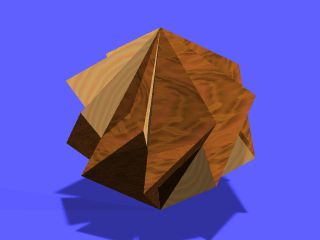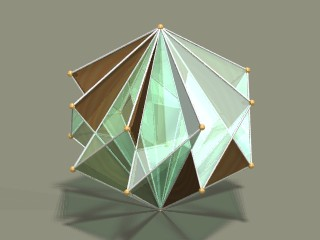Some nonconvex deltahedra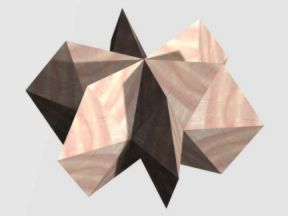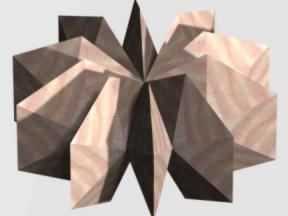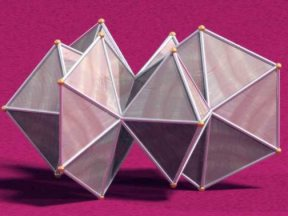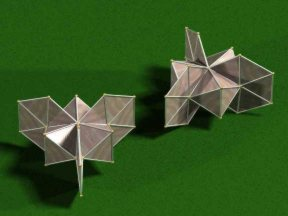The images at left are nonconvex "bellows deltahedra". Each is made by piecing together "pockets" made of a number of equilateral triangles. In the first image at the far left, the pockets have 8 triangles each. The pocket can take any shape from flat ("mouth opening" 120°, equatorial angle subtended 0°) to octahedral ("mouth opening" 0°, equatorial angle subtended 90°) Thus any number of these pockets from 4 up can be joined. As we see, the "mouth opening" is rather close to 120° even for six pockets.

The deltahedron in the bottom left image has 10 triangles in each pocket, and the pockets can be any shape from flat to pentagonal dipyramids. The dipyramid subtends an equatorial angle of 108° which does not divide 360°; four or more partially-collapsed pockets can form a deltahedron.

The two deltahedra in the center are hybrids made out of two types of pocket unit. As the larger pocket (which has the smaller "mouth") stops the smaller pocket from opening its "mouth" - and hence from reducing its equatorial angle - beyond a certain point , these are limited at least in how many of the smaller pockets they can contain.

These deltahedra are rigid (at least in theory). Each pocket is flexible, but its "mouth opening" and equatorial angle determine each other. As every pocket has the same mouth opening, every pocket also has the same equatorial angle; and this latter is thus necessarily 360°/n where n is the number of pockets. These constructions show that a deltahedron can have arbitrarily many faces meeting at a point.

This is a great model to make out of paper! Beginners can easily lay out one side of a pocket using compasses, then use a photocopier to copy onto heavy paper. If the edges of the first and last pockets are not glued together, the whole model can be folded flat for transportation.

Back to Robert Dawson's home page ............Back to Robert Dawson's raytracing page